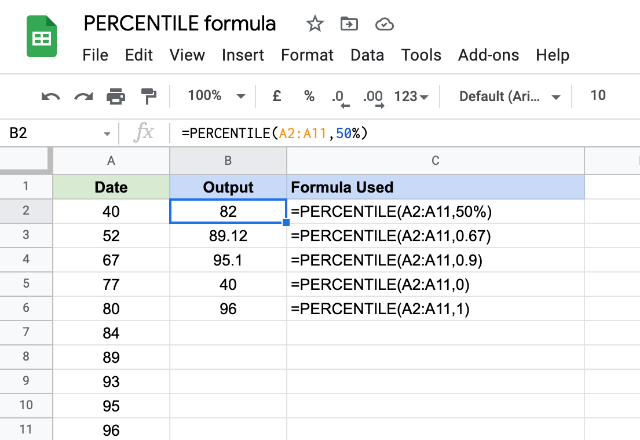# How to use the PERCENTILE formula in Google SheetsSpreadsheets are where most of us do our data analysis on. For that reason, we have many formulas and tools to aid us in that direction. And the PERCENTILE formula in Google Sheets is one such formula. It gives us the value below which the specified percentage of observations in a group fall. It essentially segregates the data points into 100 equidistant segments. So the 50th percentile, for example, would return the value placed in the 50th segment.

### Syntax

PERCENTILE(data, percentile)

• data – this is the reference to the range of cells that contains the numeric dataset we want to calculate the percentile for.
• percentile – is the percentile for which the formula calculates the value within data and returns the output. The values range from 0 to 100 percentiles and they are expressed in percentage terms. For instance, we use 0.5 (or 50%) for the 50th percentile, which incidentally returns the median value in the dataset. Similarly, we go with 0.67 (or 67%) for the 67th percentile.

### Usage: PERCENTILE formula in Google Sheets

Please consider the following screenshot, that demonstrates the practical application of this formula. We have a numeric dataset in column A. And we have a few percentile calculations as illustrated in column B.The first example is calculating the 50th percentile – meaning that we see the median value of the dataset. It may so happen that the output may not correspond to any single value in the dataset. And that is what happened here. The second and third examples calculate the 67th and 90th percentiles respectively. And they don’t correspond to any single data point either.

Now one might begin to wonder, what would a 0th and 100th percentile look like. So, we’ve tried them too, in the fourth and fifth examples. And it is no surprise that the 0th percentile displayed the lowest value in the dataset, while the 100th percentile got us the highest value.

You may have noticed the data is sorted in ascending order – that is done just for the purposes of our quick understanding. However, in real life scenarios, it is not necessary to have a sorted data for input.